• 最近有个朋友问起的一个问题，给定一个荷质比和保留时间构成的平面，想看下哪些化合物落在这个平面里面。假如下面一个平面，哪些平面里？哪些平面外？怎么判断呢？最简单的方法是目测！如果采...


最近有个朋友问起的一个问题，给定一个荷质比和保留时间构成的平面，想看下哪些化合物落在这个平面里面。假如有下面一个平面，哪些点在平面里？哪些点在平面外？怎么判断呢？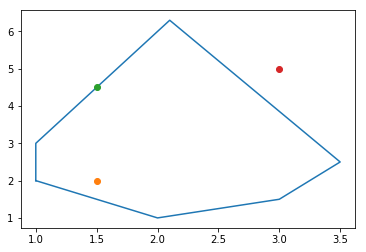最简单的方法是目测！如果采用程序，其中一个方法是光线投射算法，如下图所示：从任意位置画一条到目标点的水平线，计算该水平线进出平面边界的次数，如果是偶数，则点在平面外；如果是奇数，则点在平面内。点正好落在定点或边界时会出现特殊的判断。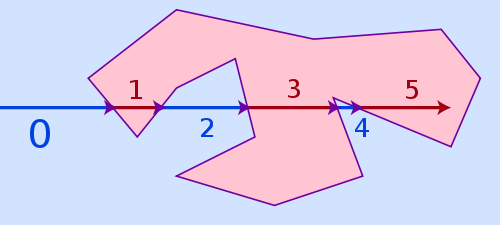我们自己构造一个多边形，绘制几个点：# (1.5,2)bounding_points = [(1,2),(2,1),(3,1.5),(3.5,2.5),(2.1,6.3),(1,3)]
bounding_box_positions = [(1.5,2), (1.5,4.5), (3,5)]如下图plotPolygenAndPoints(bounding_points, bounding_box_positions)加上从左侧发过来的光线plotPolygenAndPoints(bounding_points, bounding_box_positions, ray=True)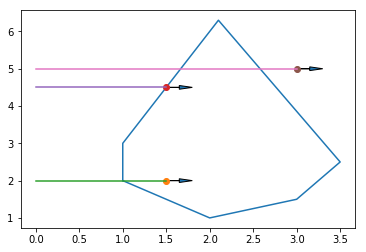原始函数不能区分部分边界上的点Stack Overflow上提供了一个函数polygon_ray_casting (代码见文末)实现ray casting算法，被转换成了各个程序语言的版本。测试下这个函数，一个边界点(1.5,4.5)计算为在平面内，另一个边界点(2.5,1.25)却未落在边界内。bounding_box_positions = [(1.5,2), (1,1.25),(1.5,4.5), (3,3),(4,3.1),(2.5,1.25),(3,5),(1.0,2),(3.5,2.5)]
bounding_box_positions = sorted(bounding_box_positions, key=lambda x:x)
polygon_ray_casting(bounding_points, bounding_box_positions)[(3, 3), (3.5, 2.5)]关于这个结果在评论区也有讨论，函数设计的目的不同，行为也会不同。在公共平台找到的代码和方法是给我们提供思路的，有些代码可以直接用，有些代码需要修改后用。使用前，要注意其使用条件，测试后再用于自己的数据。修改后的函数可处理边界点增加边界点的判断、定点的判断增加边界点的判断、定点的判断测试凸多边形# 默认落在边界也算polygon_ray_casting_modi(bounding_points, bounding_box_positions)[(1.0, 2), (3, 3), (3.5, 2.5)]plotPolygenAndPoints(bounding_points, bounding_box_positions)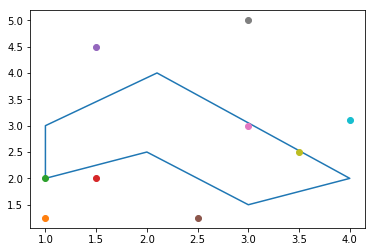# 落在边上不算在内polygon_ray_casting_modi(bounding_points, bounding_box_positions, edgeAsInside=False)[(1.5, 2), (3, 3)]测试凹多边形# (1.5,2)bounding_points = [(1,2),(2,2.5),(3,1.5),(4,2),(2.1,4),(1,3)]
bounding_box_positions = [(1.5,2), (1,1.25), (1.5,2.5), (3,1.5), (3,3),(4,3.1), (1,2.1), (3.5,1.75)]
bounding_box_positions = sorted(bounding_box_positions, key=lambda x:x)
polygon_ray_casting_modi(bounding_points, bounding_box_positions)[(1, 2.1), (1.5, 2.5), (3, 1.5), (3, 3), (3.5, 1.75)]plotPolygenAndPoints(bounding_points, bounding_box_positions)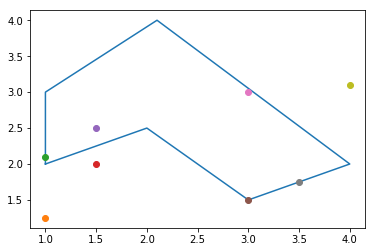测试多种六边形bounding_points = [(1,3),(2,3),(3,2),(2,1),(1,1),(0,2)]
bounding_box_positions = [(-1,3), (1.5,2.9), (1.5,3),(0,2),(1,3),(0.5,1.5),(2.5,1.5), (2.5,3),(0,1.5),(1,1.1)]
bounding_box_positions = sorted(bounding_box_positions, key=lambda x:x)
polygon_ray_casting_modi(bounding_points, bounding_box_positions)[(0, 2), (0.5, 1.5), (1, 3), (1, 1.1), (1.5, 2.9), (1.5, 3), (2.5, 1.5)]plotPolygenAndPoints(bounding_points, bounding_box_positions)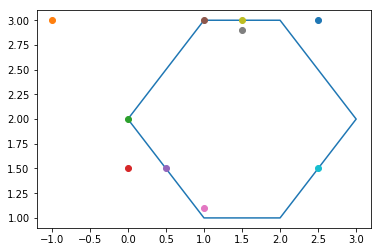# (1.5,2)bounding_points = [(1,3),(2,3),(2,2),(3,2),(3,1),(1,1)]
bounding_box_positions = [(1.5,2), (1.5,3), (1,3), (3,1.25), (3,3)]
polygon_ray_casting_modi(bounding_points, bounding_box_positions)[(1.5, 2), (1.5, 3), (1, 3), (3, 1.25)]plotPolygenAndPoints(bounding_points, bounding_box_positions)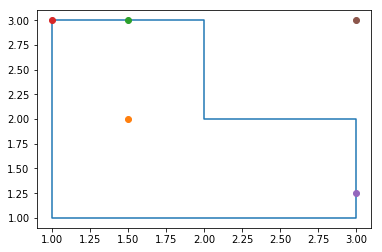# (1.5,2)bounding_points = [(1,2),(3,2),(3,3),(4,3),(4,1),(1,1)]
bounding_box_positions = [(1.5,2), (1,1.25), (3,3),(4,3.1)]
polygon_ray_casting_modi(bounding_points, bounding_box_positions)[(1.5, 2), (1, 1.25), (3, 3)]plotPolygenAndPoints(bounding_points, bounding_box_positions)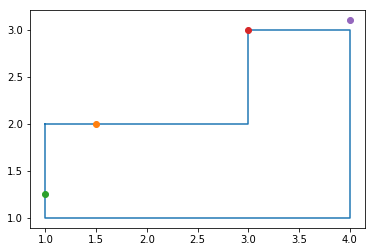for i in bounding_box_positions:
print(i)
print(wn_PnPoly(i, bounding_points))(1.5, 2)
0
(1, 1.25)
-1
(3, 3)
0
(4, 3.1)
0用到的绘图函数import matplotlib.pyplot as pltdef plotPolygenAndPoints(bounding_points, bounding_box_positions, ray=False):
coord = bounding_points[:]
coord.append(coord) #repeat the first point to create a 'closed loop'

xs, ys = zip(*coord) #create lists of x and y values

plt.figure()
plt.plot(xs,ys)
for x,y in bounding_box_positions:
plt.plot(x,y,'o')        if ray:
plt.plot((0,x),(y,y))

plt.show()用到的判断函数def polygon_ray_casting(bounding_points, bounding_box_positions):
# https://wrf.ecse.rpi.edu/Research/Short_Notes/pnpoly.html
# https://stackoverflow.com/questions/217578/how-can-i-determine-whether-a-2d-point-is-within-a-polygon
# Arrays containing the x- and y-coordinates of the polygon's vertices.
vertx = [point for point in bounding_points]
verty = [point for point in bounding_points]    # Number of vertices in the polygon
nvert = len(bounding_points)    # Points that are inside
points_inside = []    # For every candidate position within the bounding box
for idx, pos in enumerate(bounding_box_positions):
testx, testy = (pos, pos)
c = 0
for i in range(0, nvert):
j = i - 1 if i != 0 else nvert - 1
if( ((verty[i] > testy ) != (verty[j] > testy))   and
(testx < (vertx[j] - vertx[i]) * (testy - verty[i]) / (verty[j] - verty[i]) + vertx[i]) ):
c += 1
# If odd, that means that we are inside the polygon
if c % 2 == 1:
points_inside.append(pos)    return points_inside最终用的函数def between(a,x,y,equal=True, epsilon=0.000001):
if equal:        return (x<=a<=y) or (x>=a>=y) or (abs(a-x)<epsilon) or (abs(a-y)<epsilon)    else:        return (x<a<y) or (x>a>y)def polygon_ray_casting_modi(bounding_points, bounding_box_positions, edgeAsInside=True, epsilon=0.000001):
# https://wrf.ecse.rpi.edu/Research/Short_Notes/pnpoly.html
# https://stackoverflow.com/questions/217578/how-can-i-determine-whether-a-2d-point-is-within-a-polygon
# Arrays containing the x- and y-coordinates of the polygon's vertices.
vertx = [point for point in bounding_points]
verty = [point for point in bounding_points]    # Number of vertices in the polygon
nvert = len(bounding_points)    # Points that are inside
points_inside = []    # For every candidate position within the bounding box
for idx, pos in enumerate(bounding_box_positions):
testx, testy = (pos, pos)

inside = False
for i in range(0, nvert):
j = i - 1 if i != 0 else nvert - 1

# Point on horizontaol edge
if (verty[i] == testy ) and (verty[j] == testy) and between(testx, vertx[i],vertx[j]):
inside = edgeAsInside                break

# corner point
if (((verty[i] == testy) and (vertx[i] == testx)) or ((verty[j] == testy) and (vertx[j]==testx))):
inside = edgeAsInside                break

if between(testy, verty[i], verty[j]):                if(testy==verty[i]<=verty[j]) or (testy==verty[j]<=verty[i]):                    continue
c = (vertx[i]-testx)*(verty[j]-testy) - (vertx[j]-testx)*(verty[i]-testy)                # print(c)
if c==0 or abs(c) < epsilon:
inside = edgeAsInside                    break
if (verty[i]<verty[j]) == (c>0):
inside = not inside        # If odd, that means that we are inside the polygon
#print(inside)
if inside:
points_inside.append(pos)    return points_inside参考https://stackoverflow.com/questions/8721406/how-to-determine-if-a-point-is-inside-a-2d-convex-polygon/23223947#23223947https://github.com/mikolalysenko/robust-point-in-polygonhttps://wrf.ecse.rpi.edu//Research/Short_Notes/pnpoly.htmlhttps://stackoverflow.com/questions/217578/how-can-i-determine-whether-a-2d-point-is-within-a-polygon?page=1&tab=votes#tab-tophttps://en.m.wikipedia.org/wiki/Point_in_polygon完整代码 https://gitee.com/ct5869/shengxin-baodian往期精品(点击图片直达文字对应教程)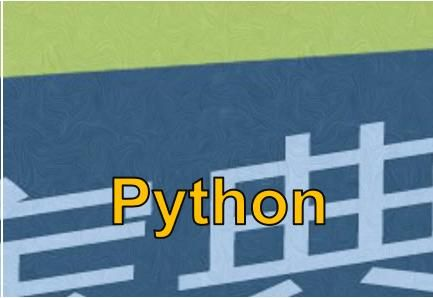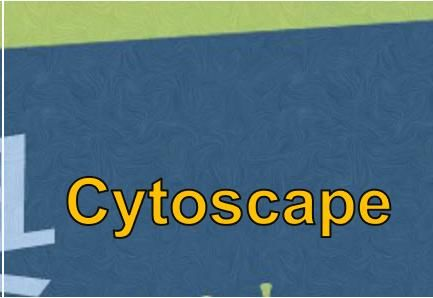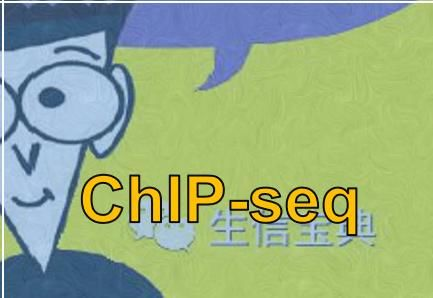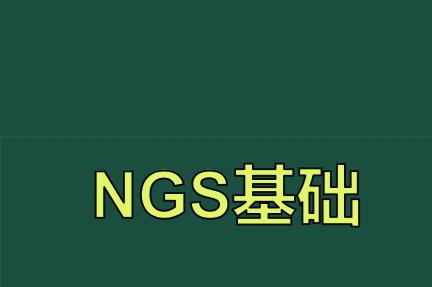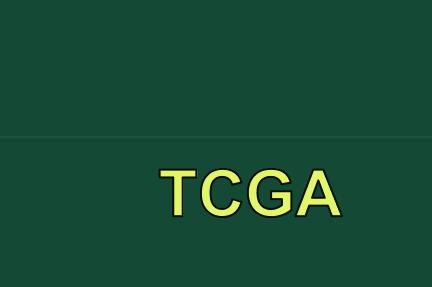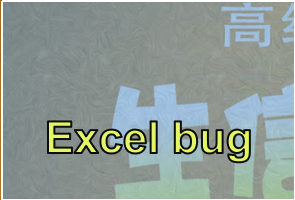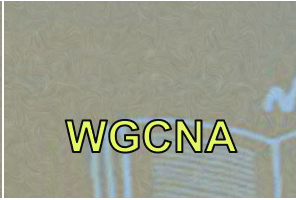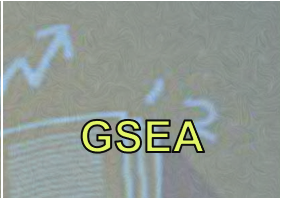机器学习后台回复“生信宝典福利第一波”或点击阅读原文获取教程合集展开全文css html js qt mapreduce
• REFERENCE:http://blog.csdn.net/happy__888/article/details/399467 原题参考 mmmcd 超超 在这帖子当中的讨论 ... N任意圆相交求公共面积的问题 必须注意到如下 ：凸体和凸体相交
REFERENCE:http://blog.csdn.net/happy__888/article/details/399467
原题参考 mmmcd 超超 在这个帖子当中的讨论http://community.csdn.net/Expert/topic/4075/4075074.xml?temp=.4154627
N个任意圆相交求公共面积的问题
必须注意到如下
一：凸体和凸体相交依然是凸体，多元相交后也是凸体
二：多元相交结果，必然是由圆弧所组成，
三：最终的相交面积为各个子圆弧的弓形面积 + 内部凸多边形的面积

要解决这个问题，要解决下面几个问题：
第一个：圆弧的表达方法
Arc(圆心位置，半径，开始角度和结束角度，P1， P2)
p1，p2是两个交点的位置
交点表达：
P(x, y, c1, c2)  : 必然是两个 圆交出来的
第二个： 建立同圆的圆弧相交算法
只有两个圆弧是同一个圆上的时候，才用此算法
算法运算的结果是生成一组圆弧，他们的圆心，半径相同，其他的参数不同
第三个： 任意两圆相交结果的圆弧表达方法
任意两个圆做相交判断，结果有三种：
相  交：得到组成相交结果的两个圆弧
不相交：得到两个空的圆弧
包  含：得到一个空圆弧，一个满圆弧
有了这三个算法，n个圆相交的解决方案如下
第一步：
n个圆两两执行相交计算，把结果用圆弧表示，执行结果是一个N×N的表格
执行次数是n*(n-1)/2, 每次运算填充表的两个位置
第一个圆    第二个   第三个   第四个  .....  第n个
第一个圆      *        arc12    arc13    arc14   .....   arc1n
第二个圆   arc21        *       arc23    arc24   .....   arc2n
第三个圆   arc31       arc32    *        arc24   .....   arc2n
第四个圆   arc41       arc42    arc23    *               arc2n
........     ................................................
第n个圆    arcn1      arcn2     arcn3    arcn4   .....     *
在这个表格当中，每次运算填充的是arcij和arcji两个位置
比如,第3个和第4个圆相交，结果写入arc34和arc43
arc34是相交后的，第三个圆上的圆弧， arc43则是相交后的第四个圆上的圆弧
如果在执行这个运算过程当中
arcij 和 arcji都是空圆弧，那么证明圆i和圆j不相交，全体的交集必然是空，可以停止算法了
arcij 和 arcji一个空，一个满。那么空的圆可以抛弃当作不存在，因为他们之间是包含关系

第二步
对表的每行做同圆的圆弧的相交运算，得到的最终结果依然是多段圆弧
相交的结果，可能是空，但是依然是有效的答案
第三步，串连所有的圆弧
从第二步计算得到的结果当中，随意找到一个不空的圆弧，从中找到任意一个圆弧，开始串连
串连的方法：
总面积S为0， 串连点队列L为空
从表当中选择一个圆，这个圆的表内同圆圆弧相交计算后得到的圆弧数组不空，从这个数组当中任意取得一个圆弧，作为当前圆弧
while(1)
{
把当前圆弧的P1点加入串连顶点队列L当中
计算这个圆弧的弓形面积，加入总面积S当中
if ( 当前圆弧的P2点是顶点队列L的第一个点) {
是跳出循环，结束串连
}
根据P2（x，y，c1，c2）点，找到它和另外一个圆的交点对应的圆弧，并把该圆弧作为当前的圆弧， 继续循环
}
第四步, 计算面积
在执行完成第三步之后， 串连点队列L就是N个圆相交部分的凸多边形的顶点队列，计算它的面积加入到S当中就完成了任务

展开全文algorithm 算法
• 一个平面坐标系上，矩形，它们的边分别平行于X和Y轴。 其中，矩形A已知， ax1(左边), ax2（右边）, ay1（top的纵坐标）, ay2（bottom纵坐标）. 矩形B，类似，就是 bx1, bx2, by1, by2。这些值都是整数就OK...
在一个平面坐标系上，有两个矩形，它们的边分别平行于X和Y轴。其中，矩形A已知， ax1(左边), ax2（右边）, ay1（top的纵坐标）, ay2（bottom纵坐标）. 矩形B，类似，就是 bx1, bx2, by1, by2。这些值都是整数就OK了。要求是，如果矩形没有交集，返回-1， 有交集，返回交集的面积。int area(rect const& a, rect const& b){...}
typedef struct rect {
int x;	//x:x1 x:x2
int y;	//y:y2 y:y1
} Rect;

int area(rect const& a, rect const& b) {
int difX = min(a.x, b.x) - max(a.x, b.x);
int difY = min(a.y, b.y) - max(a.y, b.y);
return (difX < 0 || difY < 0) ? -1 : difX * difY;
}
展开全文• // Optional，这设置可以选定结果平面展示的是分割掉的还是分割剩下的。 seg . setOptimizeCoefficients ( true ) ; // Mandatory-设置目标几何形状 seg . setModelType ( pcl : : SACMODEL_PLANE ) ...
#include <pcl/io/pcd_io.h>
#include <pcl/point_cloud.h>
#include <pcl/point_types.h>
#include <pcl/visualization/pcl_visualizer.h>
#include <pcl/filters/extract_indices.h>
#include <pcl/segmentation/progressive_morphological_filter.h>
#include <pcl/segmentation/sac_segmentation.h>
#include <pcl/visualization/cloud_viewer.h>
#include <iostream>

void main()
{
pcl::PointCloud<pcl::PointXYZ>::Ptr cloud(new pcl::PointCloud<pcl::PointXYZ>());//点云对象
{
std::cerr << "ERROR: Cannot open file " << std::endl;
return;
}
//创建一个模型参数对象，用于记录结果
pcl::ModelCoefficients::Ptr coefficients(new pcl::ModelCoefficients);
//inliers表示误差能容忍的点 记录的是点云的序号
pcl::PointIndices::Ptr inliers(new pcl::PointIndices);
// 创建一个分割器
pcl::SACSegmentation<pcl::PointXYZ> seg;
// Optional，这个设置可以选定结果平面展示的点是分割掉的点还是分割剩下的点。
seg.setOptimizeCoefficients(true);
// Mandatory-设置目标几何形状
seg.setModelType(pcl::SACMODEL_PLANE);
//分割方法：随机采样法
seg.setMethodType(pcl::SAC_RANSAC);
//设置误差容忍范围，也就是我说过的阈值
seg.setDistanceThreshold(0.01);
//输入点云
seg.setInputCloud(cloud);
//分割点云，获得平面和法向量
seg.segment(*inliers, *coefficients);
//打印出法向量
std::cout << "x：" << coefficients->values << endl;
std::cout << "y：" << coefficients->values << endl;
std::cout << "z：" << coefficients->values << endl;
//平面点获取
pcl::PointCloud<pcl::PointXYZ>::Ptr clicked_points_3d(new pcl::PointCloud<pcl::PointXYZ>);
for (int i = 0; i < inliers->indices.size(); ++i)
{
clicked_points_3d->points.push_back(cloud->points.at(inliers->indices[i]));
}
//显示对象创建
boost::shared_ptr<pcl::visualization::PCLVisualizer> viewer(new pcl::visualization::PCLVisualizer("viewer"));
//全局点云添加
//将平面点标为红色添加
pcl::visualization::PointCloudColorHandlerCustom<pcl::PointXYZ> red(clicked_points_3d, 255, 0, 0);
std::string cloudName="plane";
viewer->setPointCloudRenderingProperties(pcl::visualization::PCL_VISUALIZER_POINT_SIZE, 5, cloudName);
//保持窗体，知道显示窗体退出
while (!viewer->wasStopped())
{
viewer->spinOnce(100);
}
}



展开全文• Milo Yip，游戏程序员、《游戏引擎架构》译者 ...1. 首先利用绝对值把 p - c 转移到第象限，下图显示不同象限的圆心也能映射至第象限，这不影响相交测试的结果： 2. 然后，把 v 减
• N任意圆相交求公共面积的问题必须注意到如下：凸体和凸体相交依然是凸体，多元相交后也是凸体二：多元相交结果，必然是由圆弧所组成，三：最终的相交面积为各个子圆弧的弓形面积 + 内部凸多边形的面积要解决这...算法 任务 c
• 整理的算法模板合集： ACM模板 目录1.平面扫描2.凸包Andrew算法直线两式转为一般式使用公式O(1)计算到直线距离3...平面N NN两两没有公共点的圆，i ii号圆的圆心在(xi,yi)(x_i,y_i)(xi​,yi​),半径为rir_iri
• 一个平面图G把平面分成若干连通片，这些连通片称为G的一个面或区域，G的面组成的集合用Φ表示 其中面积有限的区域称为平面图G的内部面，否则，称为外部面 Jordan曲线 条连续的，自身不交的，起点和终点重合...DMP 算法
• 二维平面n个点 让你求，在每个点消失次的情况下，剩余的最近公共点对距离，并求和。 【类型】 最近公共点对 分治法 or KD-Tree 【分析】 显然，我们只要先求出不删条件下的最近公共点对距离。 这距离...模板 分治
• 最近在做一个Unity实现的3D建模软件，其中需要在模型表面进行操作的时候，需要用到和三角形位置关系的判定算法。由于一个模型往往是几千三角片，所以这判定算法必须高效，否则会影响最终程序的整体性能。这里...
• 定义 相交于同一条直线的所有平面组成的集合。这条直线称为共线平面束的轴。 别称 1、平面束；... 2、因为两个平面要相交才有一公共的直线，所以A1、B1、C1和A2、B2、C2不成比例； 3、A1、B1、...
• 平面n两两没有公共点的圆，i号圆的圆心在(xi,yi),半径为ri，编号从1开始。求所有最外层的，即不包含于其他圆内部的圆。输出符合要求的圆的个数和编号。n 分析： 由于任意两圆都没有公共点条件，要判断...
•  (2)、当n>3时，每增加一个面，这面就要前面n-1面都相交，因为过同一点，两平面如果有一个公共点有一公共直线，这样就会把前面平面划分的空间一分为二，f(n)-f(n-1)=2(n-1),然后累加得f(n)=n^2-n+2几何
• ## 平面扫描

千次阅读 2017-11-23 21:22:25
平面扫描是用来降低算法的...扫描分2种情况，扫描到一个圆的左端时进行判断这圆是不是最外层的。判断方法就是在已经认为的最外层圆中找出纵坐标上下2端最近的2圆进行比较。 如果是扫到圆的右端则删除这圆（如果
• 今天分享关于高中数学必修2平面解析几何中的直线圆、圆圆的位置关系 知识，分别从三方面讲解，用5经典习题进行解答全过程。 1、直线圆的位置关系 2.圆圆的位置关系 2．求圆的弦长的常用方法 1、...高中数学
• 平面几何 直线角 直线 （不定义）直线向两方无限延伸，它无端。 射线
• 平面图性质及欧拉公式平面、定义二、定理平面图性质—欧拉公式、定理 平面、定义 G = <V,E>是一个无向图。 1.图G可嵌入平面：如果可以把图G的所有结点和边都画在平面上，同时除断点外连线之间没有...欧拉公式
• 　若可以在平面里画出一个图而让边没有任何交叉(边的交叉是指边的直线或弧线在它们的公共端点以外的地方相交)，则这图是平面性的。这样种画法称为这图的平面表示。 显然，证明一个图是非平面性比证明一个图是...
• 球形摄像机是种常见的外形类似椭球形的监控摄像机，街道十字路口一般都挂。 其内部一般有一颗机芯，同时三颗分别控制pan(水平) tilt...因为一个预览界面中的某个相对于预览平面是固定的，而一个预览解决相对
• 该工具用于直线绘制平面户型原型，可设置线宽；通过直线互相连接，可计算出户型内外墙线；支持从任意墙中间或两端附着新的线段作为墙线。不同宽度的墙线相会于一点也可正确的计算内外...**- 若同一个点连接了N条宽原型设计 设计 3D
• 平面n两两没有公共点的圆，i号圆的圆心在（x[i],y[i]），半径为r[i]。求所有最外层的，即不包含于其他圆内部的圆。 算法思想： 因为题目内的圆不存在相交的情况， 所以直接储存每圆的左端点和右端的x...
• 如果它的每灰度值用二进制表示，选择将这8数字用8字节来表示，如32的二进制表示是00100000，将其储存为[0 0 1 0 0 0 0 0]，则其二维图像可以理解为一个8层的三维图像，每层代表一个比特平面 对比每比特...数字图像处理
• 可以用定理(非平面图判定)... 下面介绍不可分线图平面性的判定 基础--------------------1 桥　线图G中选择回路C，则C将G分为3部分用边集表示为3种：I 回路上顶点在回路内连接的边II 回路内的顶点回路上的顶算法 c
• 平面扫描思想在ACM竞赛中的应用Jan 1, 2013说明：以下为本科挫比的比赛论文，写文章的语气都是副叫兽的调调！ 摘要: 平面扫描思想在计算几何，计算机图形学，网格计算等计算机理论领域广泛的应用。非常多的...计算几何
• 若图$G=(V, E)$存在种图形表示，使得将它华仔平面上后没有两结点重合，每条边不自身相交且美哟欧两条边在它们公共关联结点以外相交，则称$G$是具有平面性的图，简称平面图。 设$G$是平面图。若$G$的图形中由边...
• 平面图的基本概念及性质 前言： 内容来源这篇博客 原文链接 为了免去跳转麻烦，直接复制博客内容过来。 基本概念 平面图：设无向图G，若能将G画在一个平面上，使得...如果条边不是桥，那它必是两面的公共边界 桥离散数学
• 章　平面上的几何艺术 人们往往从悖论中获得思维的乐趣，而几何学的悖论就是不可能图形。如今我们已创造出数千种这样的二维图像，不断挑战我们的眼睛和思维。三角形、披萨饼、七巧板也蕴藏着无穷的变化和巧妙...算术 几何...